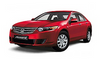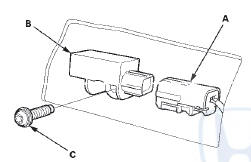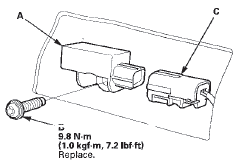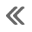Honda Accord: Rear Safing Sensor Replacement

Removal

1. Do the battery t e r m i n a l disconnection p r o c e d u r e (see page 22-91), t h e n wait at least 3 minutes before starting w o r k .

2. Remove t h e rear seat c u s h i o n (see p a g e 20-241).

3. Disconnect t h e SRS f l o o r w i r e harness 2P (4-door) or 4P (2-door) connector (A) f r om t h e rear s a f i n g sensor (B).4. Remove t h e TORX b o l t (C) u s i n g a TORX T30 b i t t h en remove t h e rear s a f i n g sensor.

Installation

1. Install t h e rear s a f i n g sensor (A) w i t h a n e w TORX bolt (B), u s i n g a TORX T30 b i t . Connect t h e SRS f l o o r w i re harness 2P (4-door) o r 4P (2-door) connector (C) t o t h e rear s a f i n g sensor.2. D o t h e b a t t e r y t e r m i n a l reconnection procedure (see page 22-91).

3. Clear a n y DTCs w i t h t h e HDS (see p a g e 24-38).

4. C o n f i rm proper SRS o p e r a t i o n : T u r n t h e i g n i t i on s w i t c h t o O N (II); t h e SRS i n d i c a t o r s h o u l d c o m e on f o r about 6 seconds and t h e n g o off.

5. Reinstall all r e m o v e d parts.Side Impact Sensor (Second) Replacement
4-Door Removal 1. Do t h e b a t t e r y t e r m i n a l d i s c o n n e c t i o n procedure (see page 22-91), t h e n w a i t at least 3 m i n u t e s before s t a r t i ng work. 2. Remove t ...Front Passenger's Weight Sensor Replacement
4-Door Removal NOTE; ╨▓╨В╤Ю Removal of the front passenger's weight sensors must be done a c c o r d i n g to Precautions and Procedures (see page 24-25). ╨▓╨В╤ЮThe front passenger' s weight ...